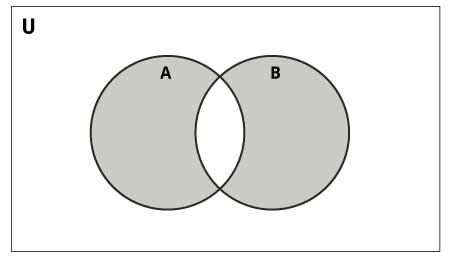# Python Set symmetric_difference_update()

The symmetric_difference_update() method finds the symmetric difference of two sets and updates the set calling it.

The symmetric difference of two sets A and B is the set of elements that are in either A or B, but not in their intersection.The syntax of `symmetric_difference_update()` is:

`A.symmetric_difference_update(B)`

## Return Value from symmetric_difference_update()

• The `symmetric_difference_update()` returns `None` (returns nothing). Rather, it updates the set calling it.

## Example: Working of symmetric_difference_update()

``````A = {'a', 'c', 'd'}
B = {'c', 'd', 'e' }

result = A.symmetric_difference_update(B)

print('A =', A)
print('B =', B)
print('result =', result)``````

Output

```A = {'a', 'e'}
B = {'d', 'c', 'e'}
result = None```

Here, the set A is updated with the symmetric difference of set A and B. However, the set `B` is unchanged.